# Power analysis

## Summary

The annotated simulation script and the annotated helper functions provide the detail that this summary leaves out.

library(knitr)
opts_chunk$set(fig.width = 12, fig.height = 12, cache = T, warning = F, message = F, cache = F) source("0_helpers.R") loadRDS("cycle_sims.rds") ## Analyse format_digits = function(x) { format(round(x, 2), nsmall = 2)} sims = cycle_sims table(round(sims$p.value - sims$p.value_KR, 10)) # exactly the same, always ## ## 0 ## 7836798 sims %>% select(-p.value_KR) -> sims print_unique = function(x) { print(unique(x)) 1 } sims %>% select(fertility_effect, nr_of_people, nr_days, dayspan, miss_window, trait_effect, predictor) %>% summarise_each(funs(print_unique)) -> x ##  0.00 0.05 0.10 0.20 0.30 0.50 0.80 ##  25 50 70 100 150 250 500 ##  2 10 30 ##  1:38 17-19,4-6 ## Levels: 1:38 17-19,4-6 ##  0 1 2 3 4 6 8 ##  0.0 0.3 ##  prc_stirn_b_m fertile_broad_m fertile_narrow_m ## Levels: prc_stirn_b_m fertile_broad_m fertile_narrow_m sims %>% group_by(fertility_effect, nr_of_people, nr_days, dayspan, miss_window, trait_effect, predictor) %>% # ,covariate_used, outcome, predictor, stopping.n summarise( usable_days = mean(usable_days, na.rm = T), not_converged = sum(is.na(p.value)), converged = sum(!is.na(p.value)), power_001 = sum(p.value <= 0.001, na.rm = T)/converged, power_01 = sum(p.value <= 0.01, na.rm = T)/converged, power_05 = sum(p.value <= 0.05, na.rm = T)/converged, power_10 = sum(p.value <= 0.10, na.rm = T)/converged, capture_95CI = sum(fertility_effect > conf.low & fertility_effect < conf.high, na.rm = T)/converged, misestimation = mean(estimate / fertility_effect, na.rm = T), misestimation_lo = quantile(estimate / fertility_effect, probs = 0.025, na.rm = T), misestimation_hi = quantile(estimate / fertility_effect, probs = 0.975, na.rm = T), abs_misestimation = mean(abs(estimate - fertility_effect), na.rm = T), misestimation_over_se = mean((estimate - fertility_effect) / std.error, na.rm = T) ) -> power ## Power analysis ### Fertile window effect .2 power %>% filter(miss_window == 0, nr_days == 30, nr_of_people %in% c(150,500), fertility_effect == 0.20, fertility_effect < 0.80, dayspan == "1:38", trait_effect == 0.3) %>% ungroup() %>% select(predictor, fertility_effect, nr_of_people,nr_days, power_01, power_05) %>% arrange(fertility_effect, predictor, nr_of_people, nr_days) %>% pander() predictor fertility_effect nr_of_people nr_days power_01 power_05 prc_stirn_b_m 0.2 150 30 0.993 0.999 prc_stirn_b_m 0.2 500 30 1 1 fertile_broad_m 0.2 150 30 0.843 0.949 fertile_broad_m 0.2 500 30 1 1 fertile_narrow_m 0.2 150 30 0.839 0.94 fertile_narrow_m 0.2 500 30 1 1 ### Fertile window effect .1 power %>% filter(miss_window == 0, nr_days == 30, nr_of_people %in% c(150,500), fertility_effect == 0.10, fertility_effect < 0.80, dayspan == "1:38", trait_effect == 0.3) %>% ungroup() %>% select(predictor, fertility_effect, nr_of_people,nr_days, power_01, power_05) %>% arrange(fertility_effect, predictor, nr_of_people, nr_days) %>% pander() predictor fertility_effect nr_of_people nr_days power_01 power_05 prc_stirn_b_m 0.1 150 30 0.445 0.689 prc_stirn_b_m 0.1 500 30 0.972 0.994 fertile_broad_m 0.1 150 30 0.199 0.414 fertile_broad_m 0.1 500 30 0.746 0.895 fertile_narrow_m 0.1 150 30 0.205 0.435 fertile_narrow_m 0.1 500 30 0.741 0.895 ### Fertile window effect .05 power %>% filter(miss_window == 0, nr_days == 30, nr_of_people %in% c(150,500), fertility_effect == 0.05, fertility_effect < 0.80, dayspan == "1:38", trait_effect == 0.3) %>% ungroup() %>% select(predictor, fertility_effect, nr_of_people,nr_days, power_01, power_05) %>% arrange(fertility_effect, predictor, nr_of_people, nr_days) %>% pander() predictor fertility_effect nr_of_people nr_days power_01 power_05 prc_stirn_b_m 0.05 150 30 0.095 0.248 prc_stirn_b_m 0.05 500 30 0.365 0.594 fertile_broad_m 0.05 150 30 0.041 0.137 fertile_broad_m 0.05 500 30 0.183 0.387 fertile_narrow_m 0.05 150 30 0.046 0.141 fertile_narrow_m 0.05 500 30 0.195 0.392 ## Number of participants versus number of days What matters for power seems to be number of days in total, no matter whether this was achieved by sampling more people or more days per person. High sample sizes should be aimed for to show generalizability and if interindividual moderators are to be tested. However, to show a main effect, it is probably considerably cheaper to ask the same people more than twice. Costs are saved on time spent on interindividual questionnaires, screening, overhead per person etc. power$n_days = power$nr_of_people * power$nr_days
power %>%
filter(fertility_effect == 0.10, dayspan == "1:38",  miss_window == 2, trait_effect == 0.3, predictor == "prc_stirn_b_m") %>%
ggplot(aes(x = n_days, y = power_01, color = factor(nr_of_people))) +
geom_text(aes( label = paste0(nr_of_people, "*", nr_days))) +
scale_x_sqrt()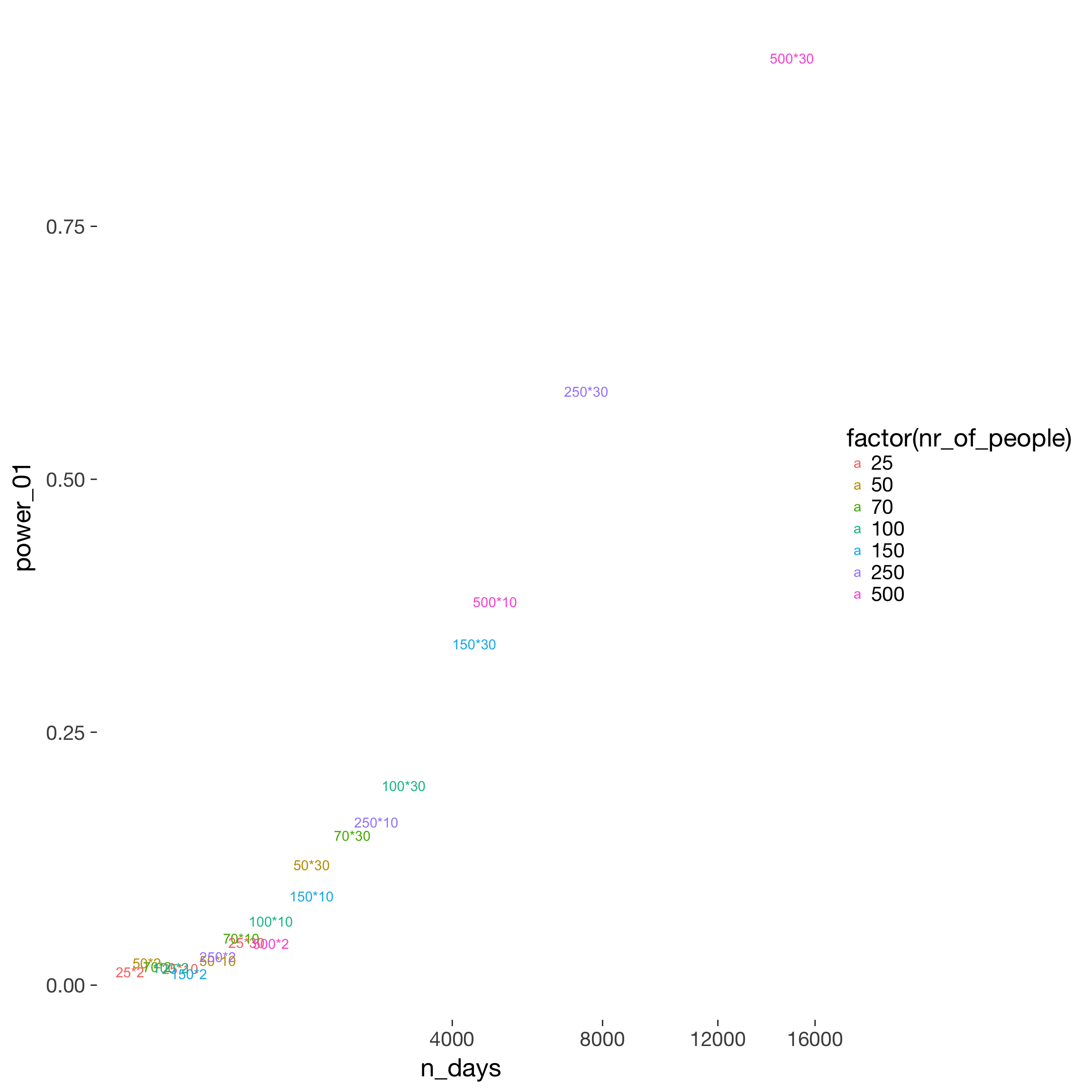power$n_days = power$nr_of_people * power\$nr_days
power %>%
filter(fertility_effect == 0.10, dayspan == "1:38",  miss_window == 2, predictor == "prc_stirn_b_m") %>%
ggplot(aes(x = n_days, y = power_05, color = factor(nr_of_people))) +
geom_text(aes( label = paste0(nr_of_people, "*", nr_days))) +
facet_wrap(~ trait_effect) +
scale_x_log10()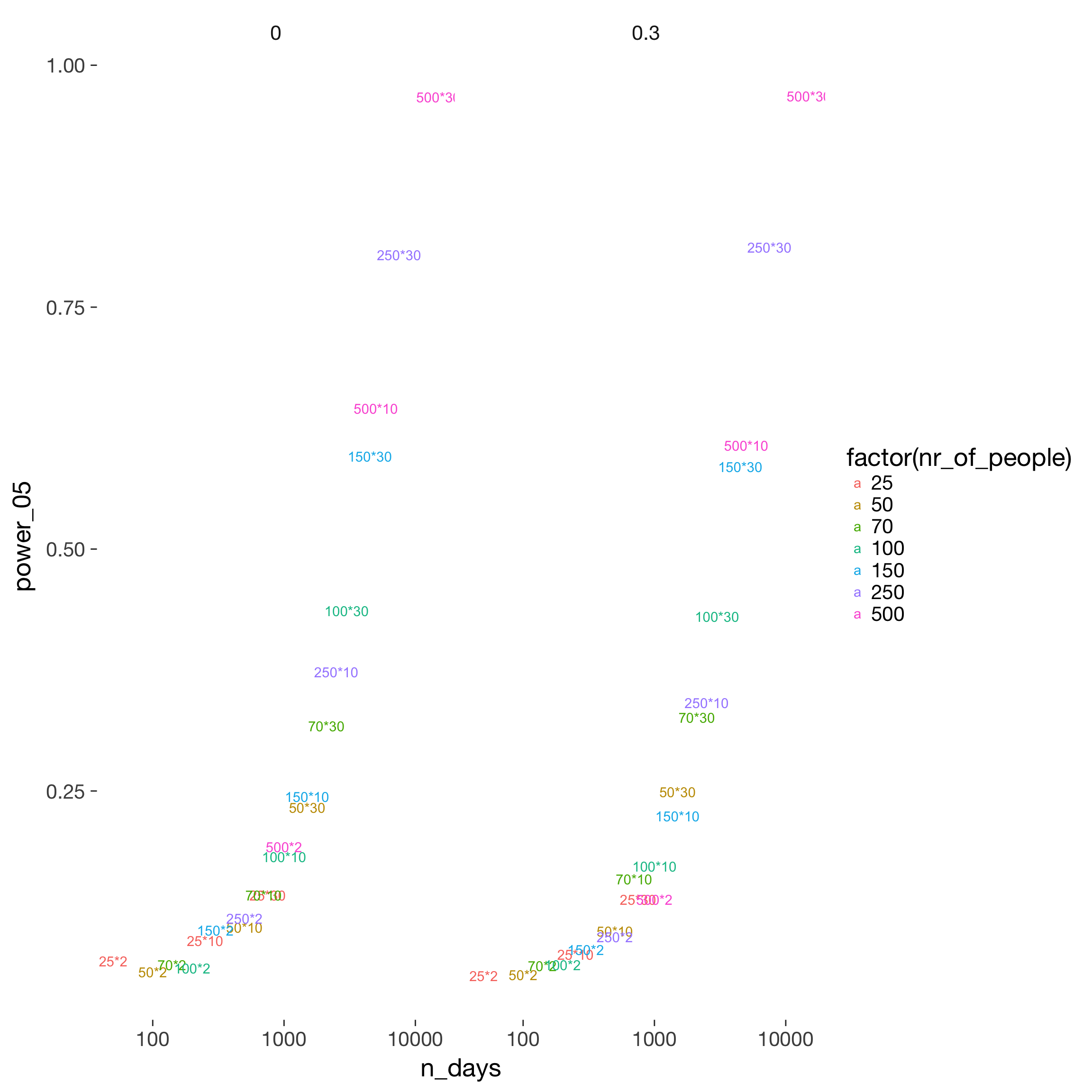power %>%
filter(fertility_effect == 0.10, dayspan == "1:38",  miss_window == 2, predictor == "prc_stirn_b_m", trait_effect == 0.3) %>%
ggplot(aes(x = n_days, y = abs_misestimation, color = factor(nr_of_people))) +
geom_text(aes( label = paste0(nr_of_people, "*", nr_days))) +
scale_x_log10() + scale_y_log10()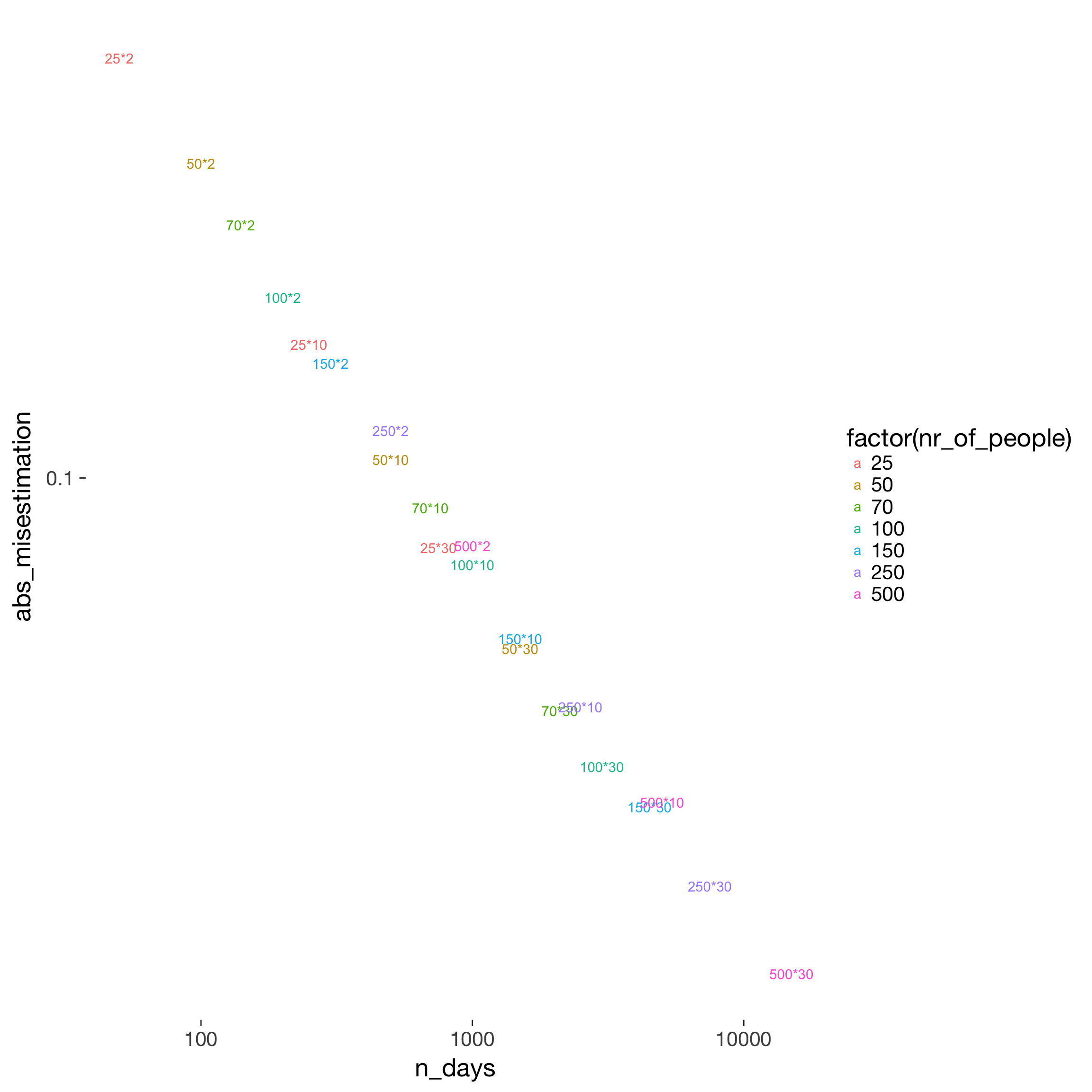## Capture percentage

I.e. when do we systematically under/overestimate. The main simulated contributor to underestimation is the number of days by which the day of ovulation is missed on average. Not simulated, but likely having a similar effect are

1. anovulatory cycles (due to e.g. stress) that are not excluded
2. infertile women in the sample
3. women with average cycle lengths other than those documented by e.g. Stirnemann
4. mis-estimation in studies that have looked at conception risk by cycle day
power %>%
filter(fertility_effect == 0.30, dayspan == "1:38", nr_of_people == 100, trait_effect == 0.3) %>%   ggplot(aes(x = predictor, y = capture_95CI)) +
geom_text(aes( label = format_digits(capture_95CI))) +
facet_grid(miss_window ~ nr_days) + theme_bw()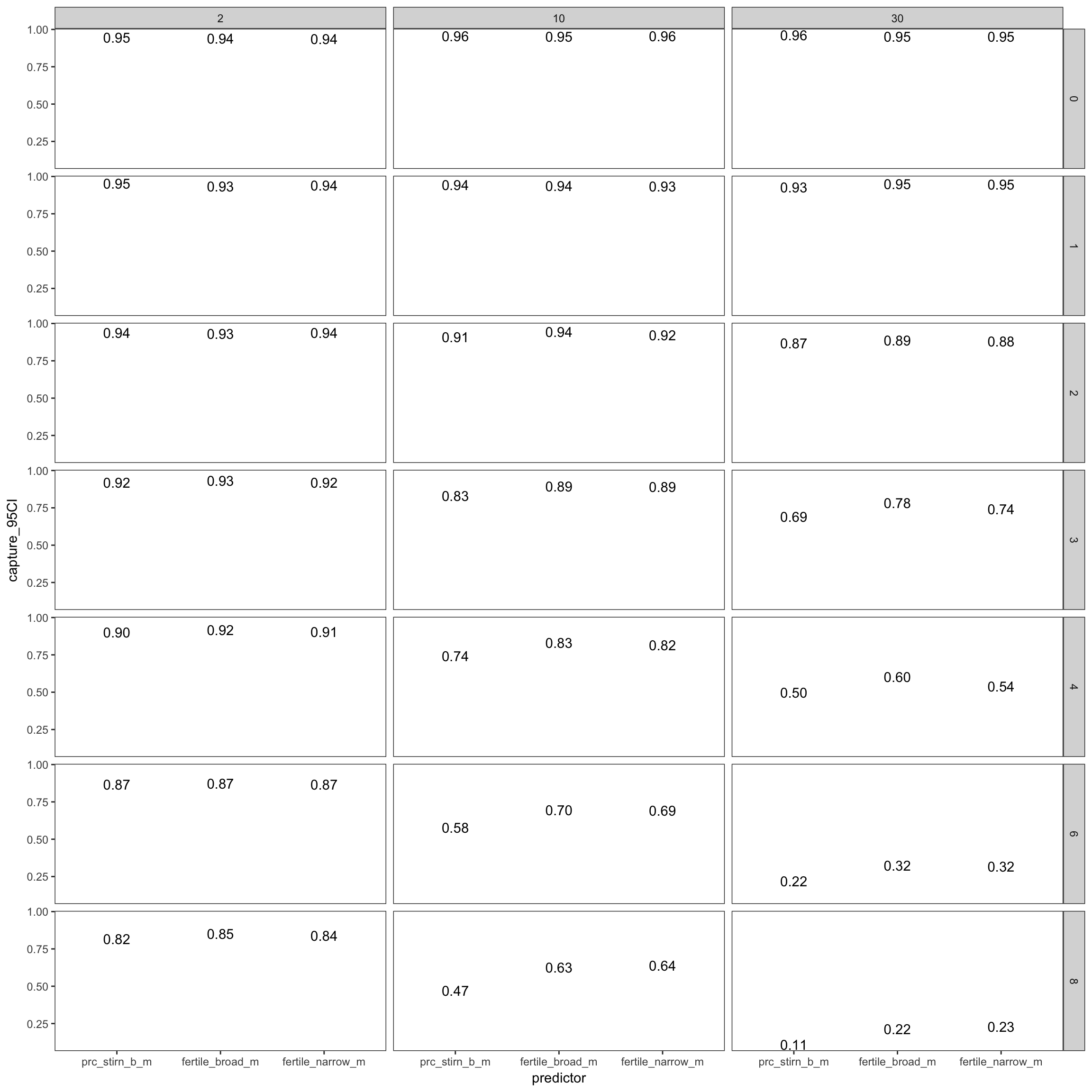### Over or under?

power %>%
filter(fertility_effect == 0.30, dayspan == "1:38", nr_of_people == 100, trait_effect == 0.3) %>%   ggplot(aes(x = predictor, y = misestimation)) +
geom_text(aes( label = format_digits(round(misestimation,2)))) +
facet_grid(miss_window ~ nr_days) + theme_bw() +
ylab("% of true effect size") +
ggtitle("Underestimation", subtitle = "left-to-right: nr. of days, top-to-bottom: avg. distance to true window")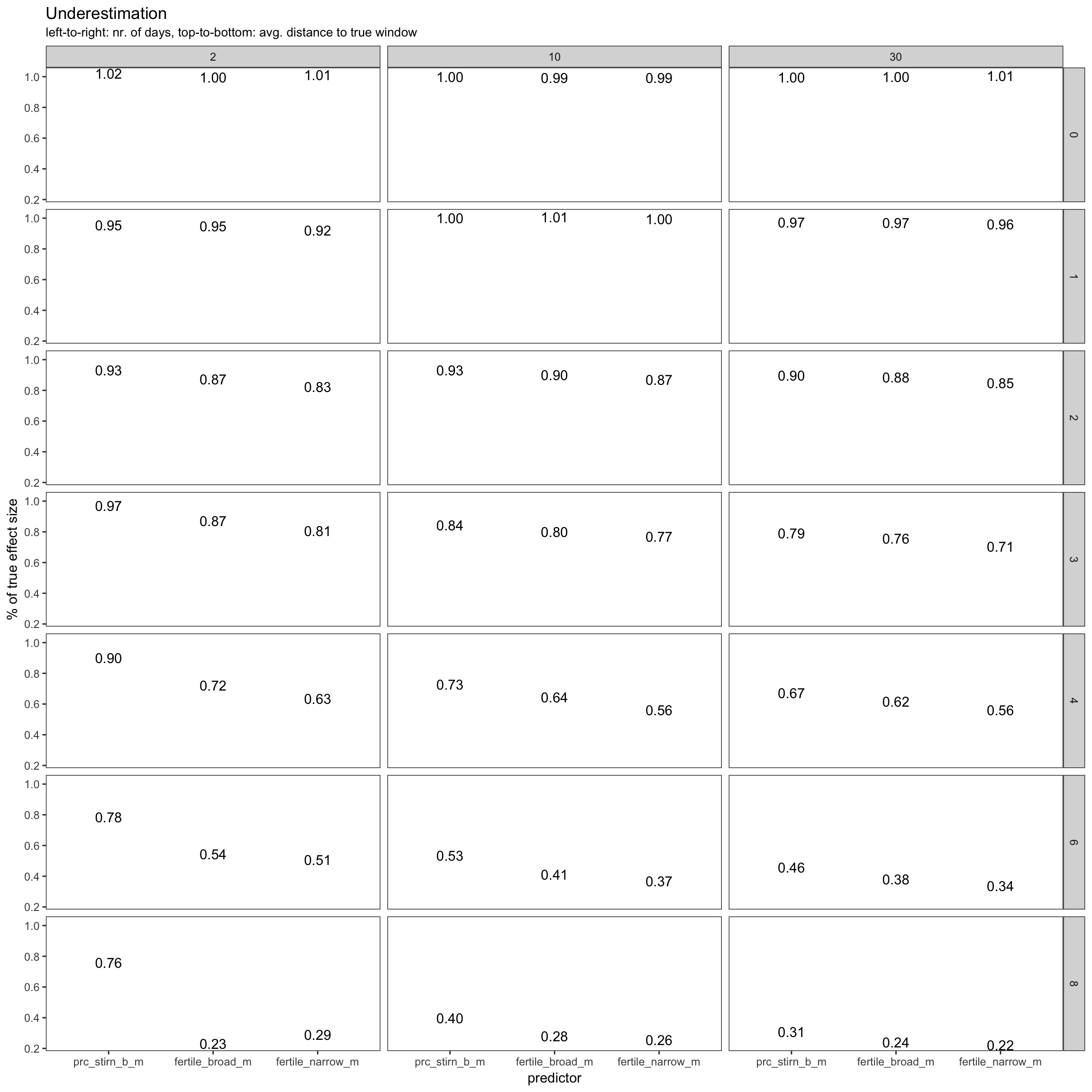for 30 days, 500 participants, missing the fertile window on average by four days

power %>%
filter(miss_window == 4, nr_days == 30, dayspan == "1:38", nr_of_people == 500, trait_effect == 0.3) %>%   ggplot(aes(x = predictor, y = misestimation)) +
geom_text(aes( label = paste0(form(misestimation), " [", form(misestimation_lo), ";", form(misestimation_hi),"]"))) +
facet_grid(fertility_effect ~ .) + theme_bw() +
ylab("% of true effect size") +
ggtitle("Underestimation", subtitle = "top-to-bottom: true fertility effect")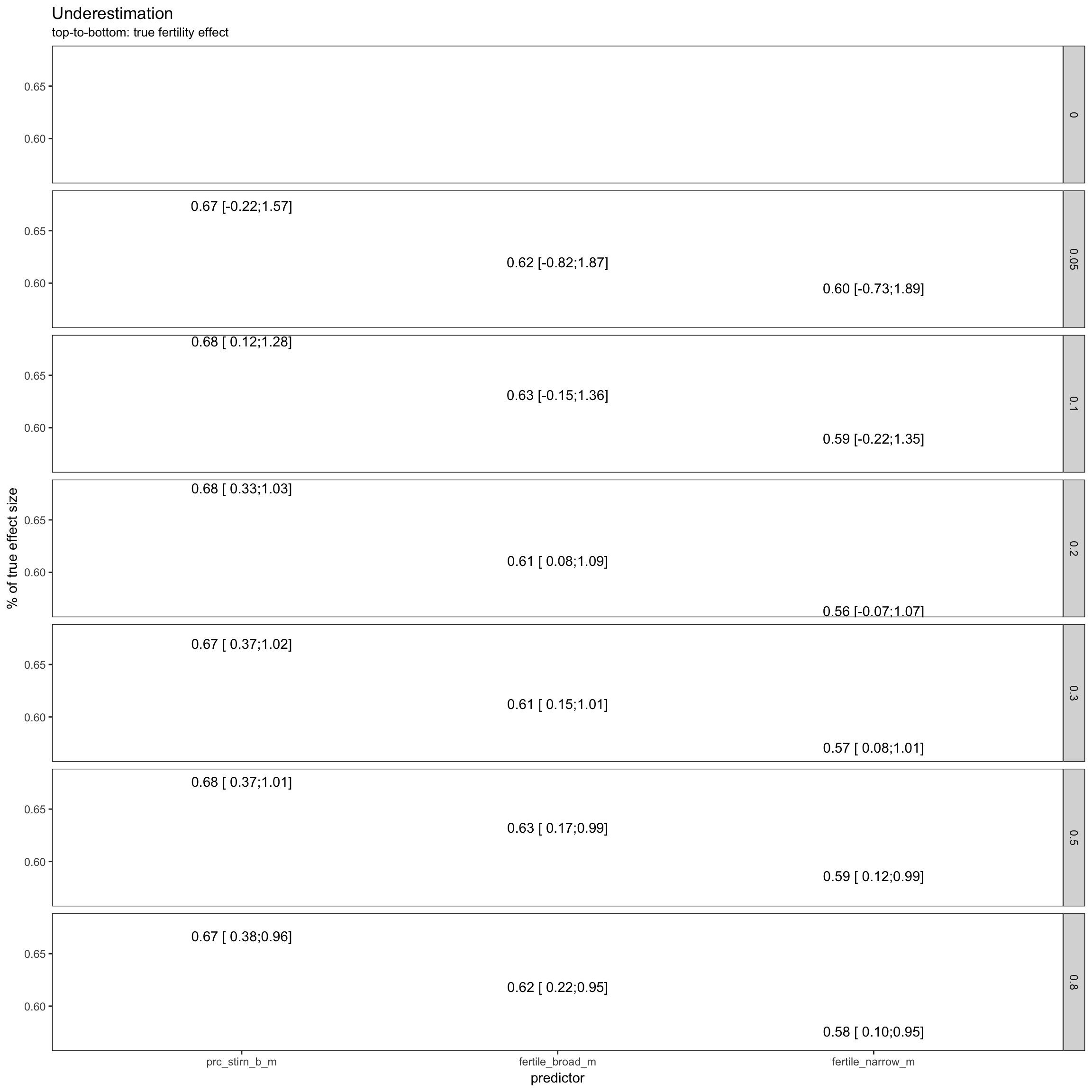power %>%
filter(miss_window == 4, nr_days == 30, dayspan == "1:38", nr_of_people == 500, trait_effect == 0.3) %>%   ggplot(aes(x = predictor, y = abs_misestimation)) +
geom_text(aes( label = format_digits(round(abs_misestimation,2)))) +
facet_grid(fertility_effect ~ .) + theme_bw() +
ylab("distance to true effect size") +
ggtitle("Underestimation", subtitle = "top-to-bottom: true fertility effect")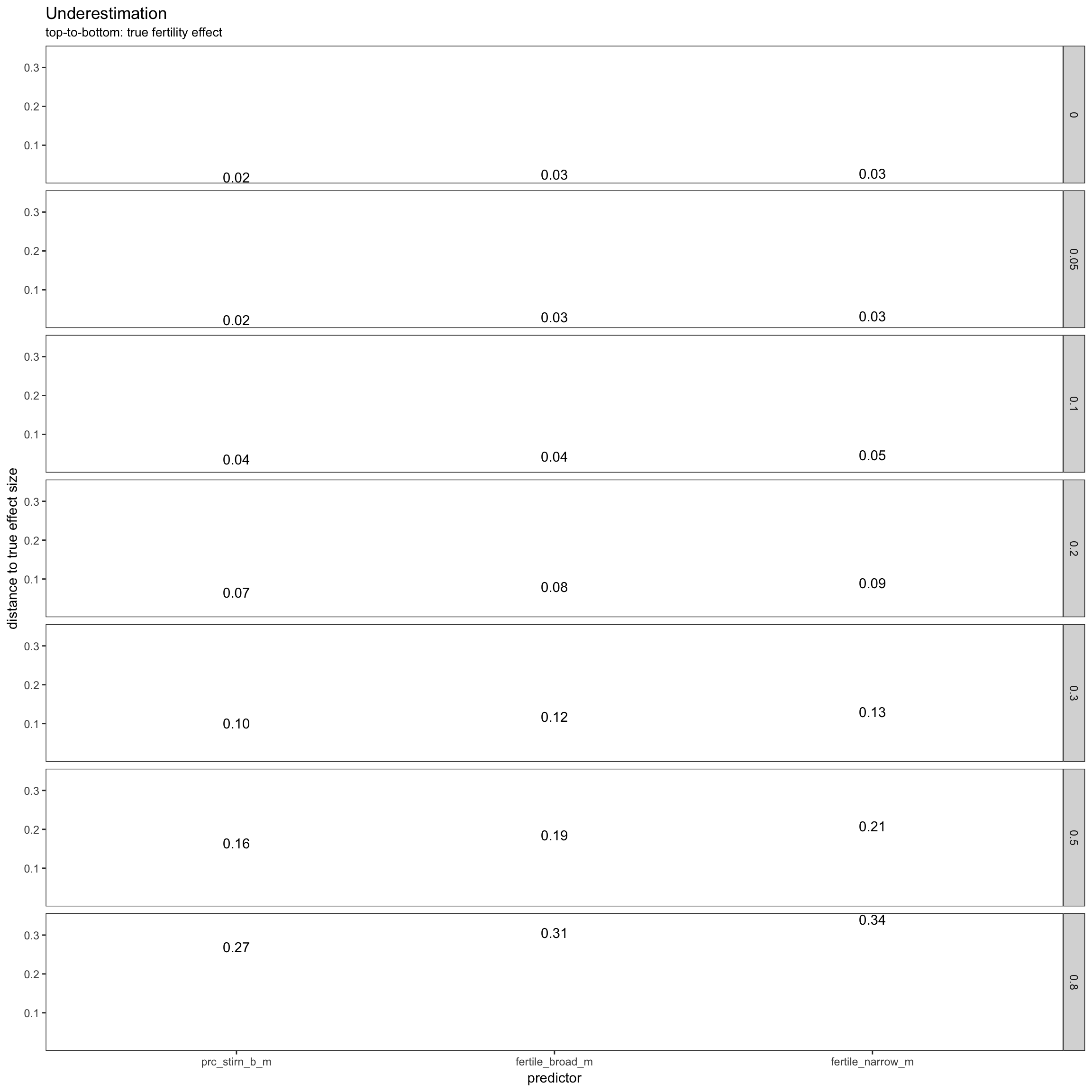sims %>%
filter(fertility_effect != 0, nr_of_people == 500, dayspan == "1:38", nr_days == 30, trait_effect == 0.3, predictor == 'prc_stirn_b_m') %>%
group_by( nr_days, dayspan, miss_window, trait_effect, predictor) %>%  # ,covariate_used, outcome, predictor, stopping.n
summarise(
misestimation = form(mean(estimate / fertility_effect, na.rm = T)),
misestimation_lo = form(quantile(estimate / fertility_effect, probs = 0.1, na.rm = T)),
misestimation_hi = form(quantile(estimate / fertility_effect, probs = 0.9, na.rm = T))
) ->
misest

pander(misest %>% ungroup() %>% select(miss_window, starts_with("misestimation")))
miss_window misestimation misestimation_lo misestimation_hi
0 1.00 0.81 1.18
1 0.98 0.79 1.18
2 0.90 0.68 1.14
3 0.79 0.54 1.06
4 0.67 0.40 0.95
6 0.46 0.14 0.79
8 0.30 -0.03 0.64